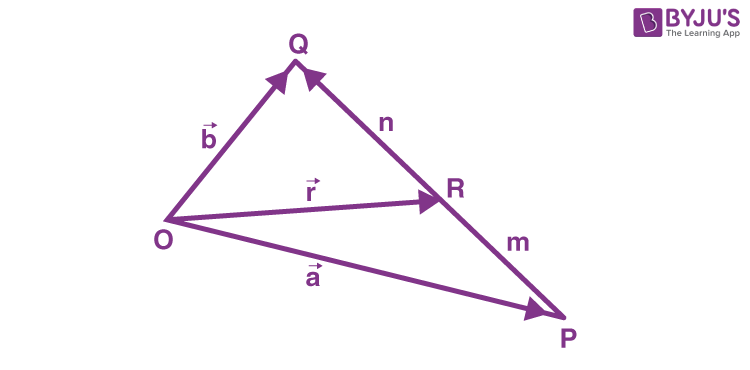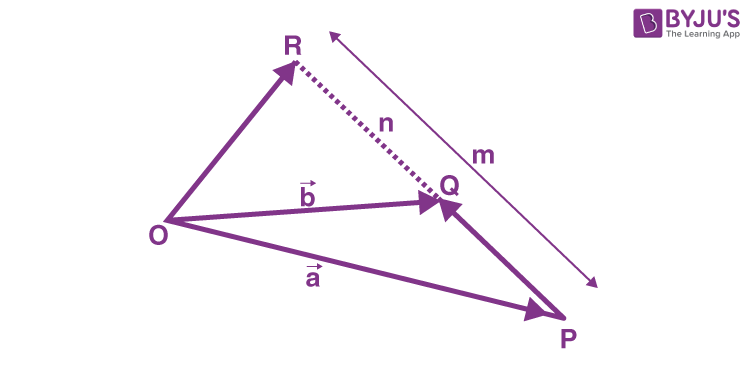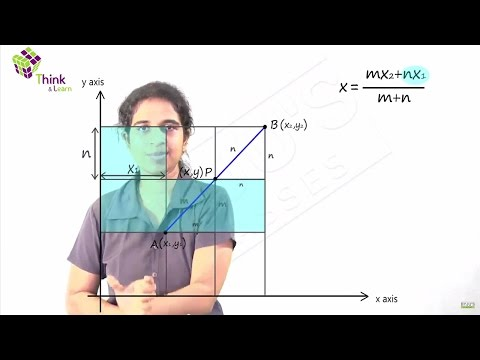Checkout JEE MAINS 2022 Question Paper Analysis : Checkout JEE MAINS 2022 Question Paper Analysis :

# Section Formula In Vector Algebra

The physical quantities that have magnitude and are directly attached to them are known as vectors. Position vectors simply denote the position or location of a point in the three-dimensional Cartesian system with respect to a reference origin. Let us see in the upcoming discussion how we can apply section formula to vectors. The concept of section formula is implemented to find the coordinates of a point dividing a line segment internally or externally in a specific ratio. To locate the position of a point in space, we require a coordinate system.

## Section Formula

If O is taken as reference origin and A is an arbitrary point in space then the vector

$$\begin{array}{l}\vec{OA}\end{array}$$
is called the position vector of the point. Let us consider two points P and Q denoted by position vectors
$$\begin{array}{l}\vec{OP}\end{array}$$
and
$$\begin{array}{l}\vec{OQ}\end{array}$$
with respect to origin O.Let us consider that the line segment connecting P and Q is divided by a point R lying on PQ. The point R can divide the line segment PQ in two ways: internally and externally. Let us consider both these cases individually.

#### Case 1: Line segment PQ is divided by R internally

Let us consider that the point R divides the line segment PQ in the ratio m: n, given that m and n are positive scalar quantities we can say that,

m

$$\begin{array}{l}\overline{RQ}\end{array}$$
= n
$$\begin{array}{l}\overline{PR}\end{array}$$

Consider the triangles, ∆ORQ and ∆OPR.

$$\begin{array}{l}\overline{RQ}\end{array}$$
=
$$\begin{array}{l}\overline{OQ}\end{array}$$
$$\begin{array}{l}\overline{OR}\end{array}$$
=
$$\begin{array}{l}\vec{b}\end{array}$$
$$\begin{array}{l}\vec{r}\end{array}$$

$$\begin{array}{l}\overline{PR}\end{array}$$
=
$$\begin{array}{l}\overline{OR}\end{array}$$
$$\begin{array}{l}\overline{OP}\end{array}$$
=
$$\begin{array}{l}\vec{r}\end{array}$$
$$\begin{array}{l}\vec{a}\end{array}$$

Therefore,

m(

$$\begin{array}{l}\vec{b}\end{array}$$
$$\begin{array}{l}\vec{r}\end{array}$$
) = n(
$$\begin{array}{l}\vec{r}\end{array}$$
$$\begin{array}{l}\vec{a}\end{array}$$
)

Rearranging this equation we get:

$$\begin{array}{l}\vec{r}\end{array}$$
=
$$\begin{array}{l}\frac{m\vec{b} + n\vec{a}}{m + n}\end{array}$$

Therefore the position vector of point R dividing P and Q internally in the ratio m:n is given by:

$$\begin{array}{l}\vec{OR}\end{array}$$
=
$$\begin{array}{l}\frac{m\vec{b} + n\vec{a}}{m + n}\end{array}$$

#### Case 2: Line segment PQ is divided by R externally

Let us consider that the point R divides the line segment PQ in the ratio m: n, given that m and n are positive scalar quantities we can say that,m

$$\begin{array}{l}\overline{RQ}\end{array}$$
= -n
$$\begin{array}{l}\overline{PR}\end{array}$$

Consider the triangles, ∆ORQ and ∆OPR.

$$\begin{array}{l}\overline{RQ}\end{array}$$
=
$$\begin{array}{l}\overline{OQ}\end{array}$$
$$\begin{array}{l}\overline{OR}\end{array}$$
=
$$\begin{array}{l}\vec{b}\end{array}$$
$$\begin{array}{l}\vec{r}\end{array}$$

$$\begin{array}{l}\overline{PR}\end{array}$$
=
$$\begin{array}{l}\overline{OR}\end{array}$$
$$\begin{array}{l}\overline{OP}\end{array}$$
=
$$\begin{array}{l}\vec{r}\end{array}$$
$$\begin{array}{l}\vec{a}\end{array}$$

Therefore,

m(

$$\begin{array}{l}\vec{b}\end{array}$$
$$\begin{array}{l}\vec{r}\end{array}$$
) = -n(
$$\begin{array}{l}\vec{r}\end{array}$$
$$\begin{array}{l}\vec{a}\end{array}$$
)

Rearranging this equation we get:

$$\begin{array}{l}\vec{r}\end{array}$$
=
$$\begin{array}{l}\frac{m\vec{b} – n\vec{a}}{m – n}\end{array}$$

Therefore the position vector of point R dividing P and Q externally in the ratio m:n is given by:

$$\begin{array}{l}\vec{OR}\end{array}$$
=
$$\begin{array}{l}\frac{m\vec{b} – n\vec{a}}{m – n}\end{array}$$

What if the point R dividing the line segment joining points P and Q is the midpoint of line segment AB?

In that case, if R is the midpoint, then R divides the line segment PQ in the ratio 1:1, i.e. m = n = 1.The position vector of point R dividing will be given as:

$$\begin{array}{l}\vec{OR}\end{array}$$
=
$$\begin{array}{l}\frac{\vec{b} + \vec{a}}{2}\end{array}$$

Go through the example problem given below to understand how to use the section formula of vectors.Example:

Consider two points A and B with position vectors and

$$\begin{array}{l}\vec{OA} = 4\vec{a} – 2\vec{b}\end{array}$$
and
$$\begin{array}{l}\vec{OB} = 2\vec{a} + \vec{b}\end{array}$$
. Find the position vector of a point C which divides the line joining A and B in the ratio 3 : 2,

(i) internally

(ii) externally.

Solution:

(i) The position vector of the point C dividing the join of A and B internally in the ratio 3 : 2 is:

$$\begin{array}{l}\vec{OC}= \frac{3(2\vec{a} + \vec{b}) + 2(4\vec{a} – 2\vec{b})}{3+2}\end{array}$$

Expanding the terms in the numerator,

$$\begin{array}{l}=\frac{6\vec{a} + 3\vec{b} + 8\vec{a} – 4\vec{b}}{5}\\=\frac{14\vec{a} – \vec{b}}{5}\end{array}$$

(ii) The position vector of the point C dividing the join of A and B externally in the ratio 3 : 2 is:

$$\begin{array}{l}\vec{OC}= \frac{3(2\vec{a} + \vec{b}) – 2(4\vec{a} – 2\vec{b})}{3-2}\end{array}$$

Expanding the terms in the numerator,

$$\begin{array}{l}=\frac{6\vec{a} + 3\vec{b} – 8\vec{a} + 4\vec{b}}{1}\\={-2\vec{a} + 7\vec{b}}\end{array}$$